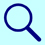PhysiologyWeb  Home  |  FAQ  |  About  |  Contact  |   - Sign InGlossary of Physiology TermsThere are 2 glossary search results for:   nernst

Definition:
An equation used to calculate the equilibrium potential (Veq.) of an ion. The equilibrium potential for an ion is also referred to as the Nernst potential for that ion. It is the membrane potential at which no net movement of the ion in question occurs across the membrane.where Veq. is the equilibrium potential, R is the universal gas constant, T is the temperature in Kelvin, z is the valence of the ionic species, F is the Faraday's constant, and [X]o and [X]i are the extracellular and intracellular, respectively, concentrations of the ion in question.

Resting Membrane Potential - Nernst Equilibrium Potential
Derivation of the Nernst Equation

Definition:
Refers to the balance of chemical and electrical gradients that act on an ion, particularly as it relates to the movement of an ion across a biological membrane (see Resting Membrane Potential - Establishment of the Membrane Potential and Resting Membrane Potential - Nernst Equilibrium Potential).

Related glossary terms/phrases: